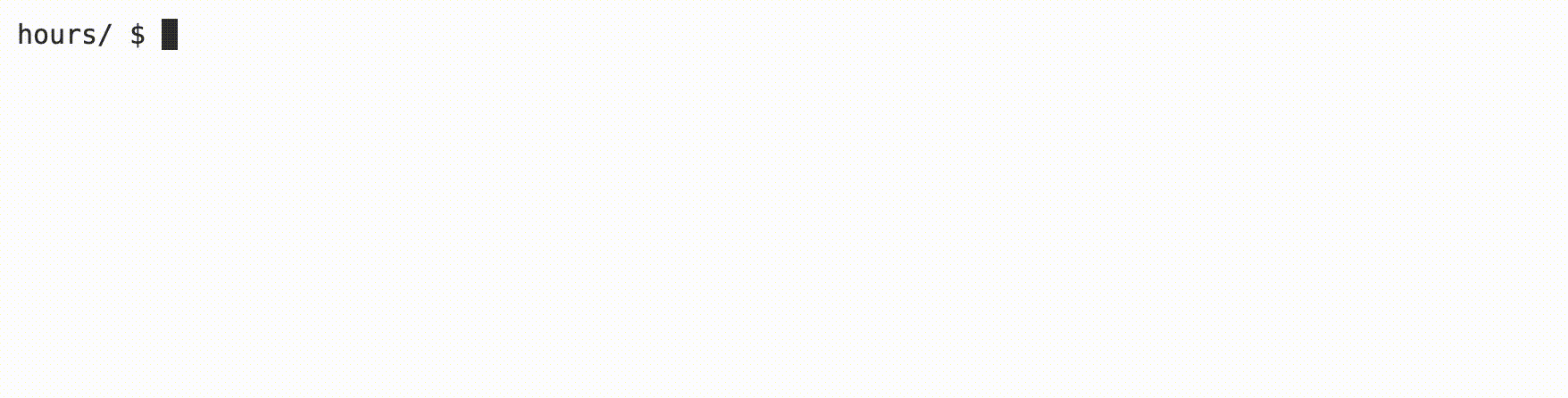# Hours

## Learning Goals

• Practice using arrays
• Using an array as a parameter to a function
• Adding values in a loop
• Integer division and type casting## Background

Suppose you’re taking CS50 (if you’re reading this you probably are!) and spending time every week on each problem set. You may be wondering how many hours you’ve spent learning computer science, on average or in total! In this program, you’ll complete a function that calculates, based on a user’s input, a total number of hours or an average number of hours across a given number of days.

• Hints
• To add up numbers in an array, you might first want to initialize a variable to zero. After, you’ll want to use a loop that adds each value in the array to that variable.
• Be sure to pay attention to what happens if you divide two ints when calculating the average!

## Demo## Getting Started

2. Click inside the terminal window and execute cd.
3. At the $ prompt, type mkdir hours 4. Now execute cd hours 5. Then copy and paste wget https://cdn.cs50.net/2022/fall/labs/2/hours.c into your terminal to download this lab’s distribution code. 6. You are to complete the function, calc_hours, which calculates both total hours and average hours, depending on the value of output. ## Implementation Details The main function prompts the user for the number of weeks a user has been taking CS50, then creates an array with as many elements. Notice that, after retrieving some data, the program prompts the user to type in either “T” or “A”—”T” should (but doesn’t yet!) print the total number of hours the user entered, while “A” should (but doesn’t yet!) print the average hours the user entered. Notice that the do while loop uses toupper to capitalize the letter that’s input before it is saved in the variable output. Then, the printf function calls calc_hours. Note the syntax involved when passing an array to a function. To complete calc_hours, first total up the hours saved in the array into a new variable. Then, depending on the value of output, return either this sum, or the average number of hours. ## Thought Question • What is the advantage of using a function to calculate hours? ## How to Test Your Code Your program should behave per the examples below. hours/$ ./hours
Number of weeks taking CS50: 3
Week 0 HW Hours: 3
Week 1 HW Hours: 7
Week 2 HW Hours: 10
Enter T for total hours, A for average hours per week: A
6.7 hours

hours/ $./hours Number of weeks taking CS50: 2 Week 0 HW Hours: 2 Week 1 HW Hours: 8 Enter T for total hours, A for average hours per week: T 10.0 hours  You can check your code using check50, a program that CS50 will use to test your code when you submit, by typing in the following at the $ prompt. But be sure to test it yourself as well!

check50 cs50/labs/2022/fall/hours


To evaluate that the style of your code, type in the following at the \$ prompt.

style50 hours.c


## How to Submit

No need to submit! This is an optional practice problem.